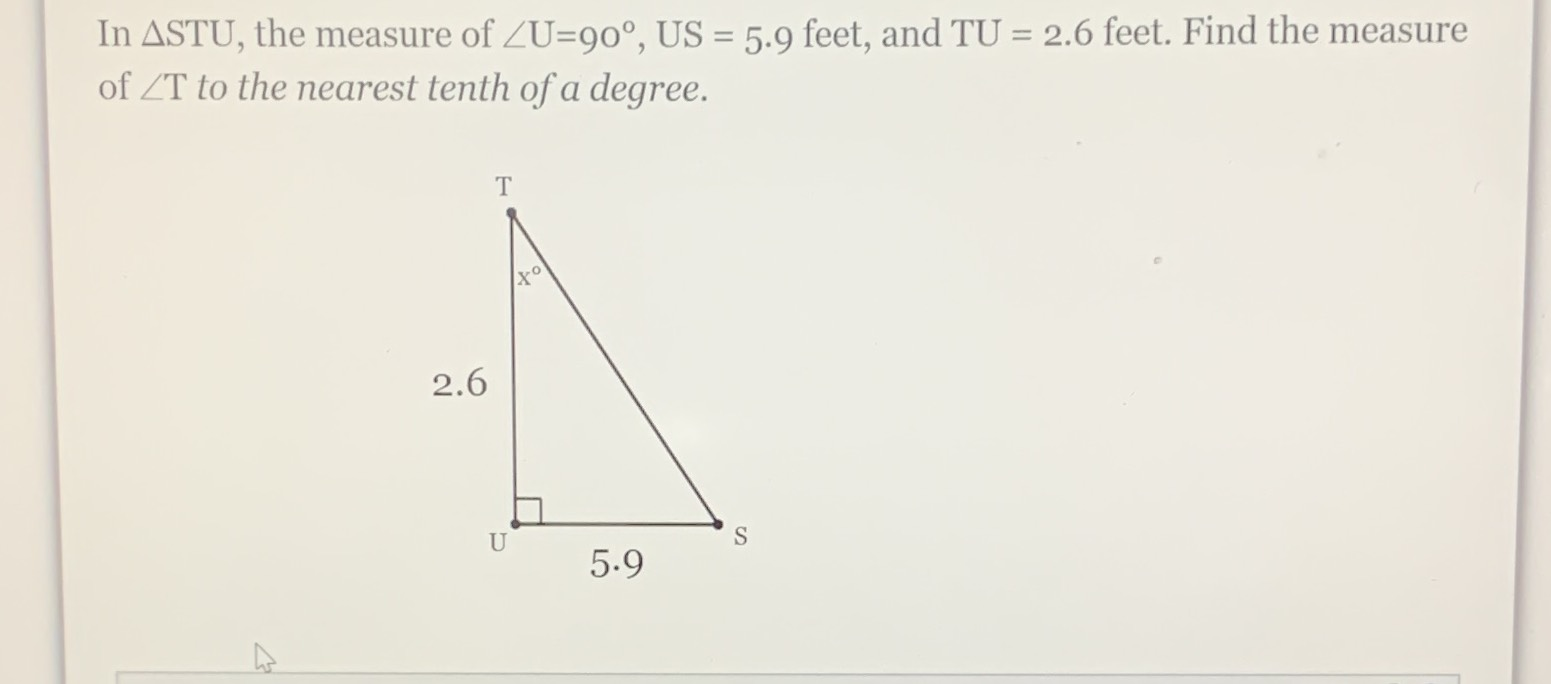Still have math questions?

Trigonometry
QuestionIn $$\triangle\mathrm{STU}$$, the measure of $$\angle\mathrm{U}=90^{\circ},$$ $$\mathrm{US}=5.9$$ feet, and $$\mathrm{TU}=2.6$$ feet. Find the measure of $$\angle\mathrm{T}$$ to the nearest tenth of a degree.

$$66.2^{\circ}$$